Smartick is a fun way to learn math!Jun26

# How to Add with Regrouping and Some Examples

In today’s post, we are going to learn how to add with regrouping.

Addition with regrouping consists of addition problems where the answer to the calculation is 10 or a bigger number.

For example, 6 + 7 = 13

The steps to solve regrouping addition problems are the following:

• If the column being added doesn’t have a carry-over (or if the number is lower than 10), write the answer under the column.
• If the column being added has a carry-over (or if the number is equal to or greater than 10), write the 1s-place numbers down under the bar and take note of the 10s-place number in the next column to add them later.

### Let’s try an example on Add with Regrouping: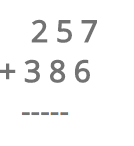Start by adding the 1s-place columns: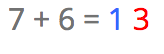This number is greater than 10, so that means you need to write 3 under the 1s-place column and the 1 (the carry-over) above the next column.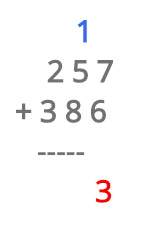Now, you just add the next column, and don’t forget about the carry-over: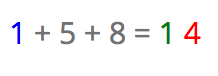This number also has a carry-over. Write the 4 down in the column of the 10s-place and bring the 1 up to the next column.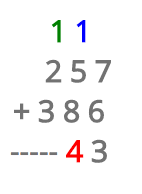Now, we just need to add the last column: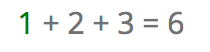The only thing left is to write that number underneath in its appropriate column: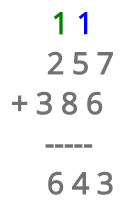And the final answer to this addition problem is 643. Did you get it right?

Do you have any questions? Check out other posts about adding and regrouping.

I hope you liked learning how to add with regrouping! If you want to keep learning more Elementary Math, try Smartick for free!